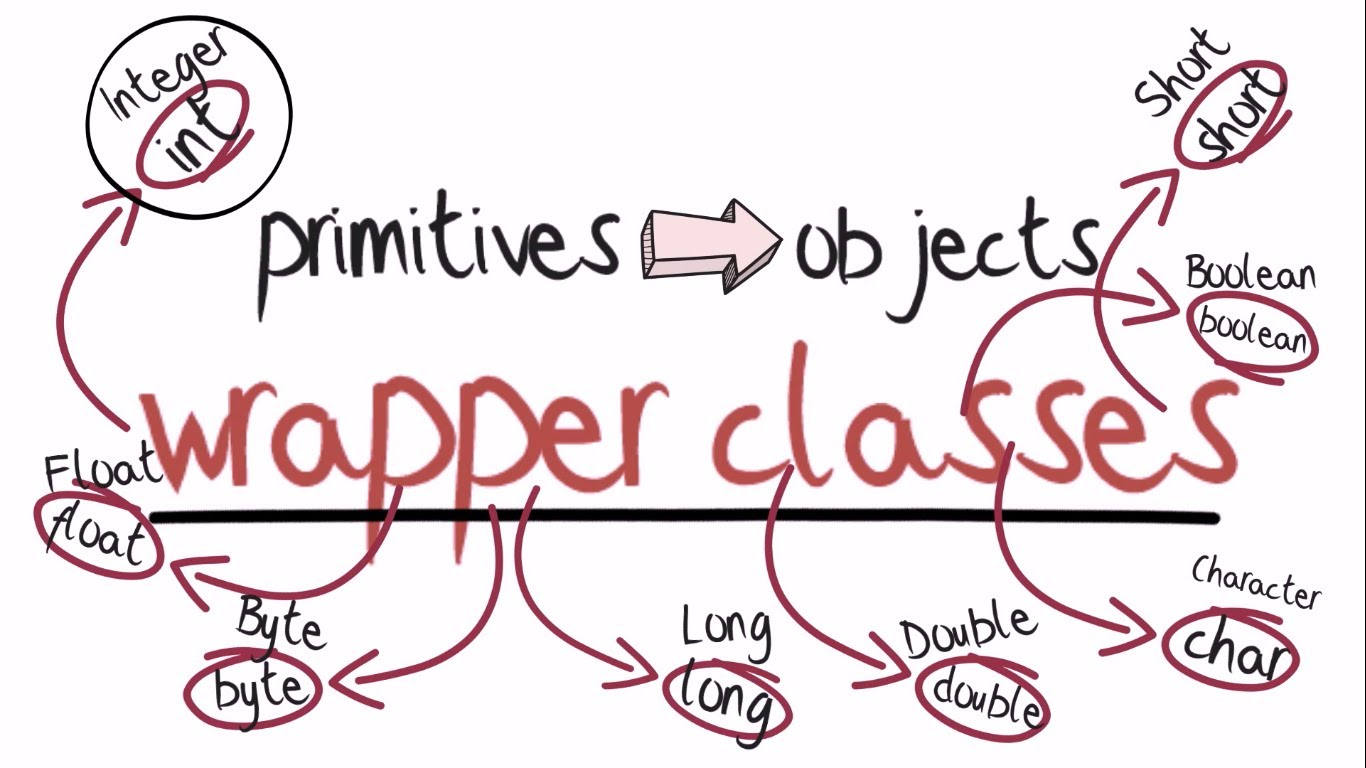Wrapper classes - Learners PoolA Wrapper class is a class whose object wraps or contains a primitive data types. When we create an object to a wrapper class, it contains a field and in this field, we can store a primitive data types.

Wrapper classes in java provides the mechanism to convert primitive into object and object into primitive. Since J2SE 5.0, autoboxing and unboxing feature converts primitive into object and object into primitive automatically. The automatic conversion of primitive into object is known as autoboxing and vice-versa unboxing.
For every primitive type there is corresponding Wrapper class is present. These classes are defined in java.lang package. The list of eight wrapper classes are given below:

 Primitive Type Wrapper class boolean Boolean char Character byte Byte short Short int Integer long Long float Float double Double
The wrapper classes in java serves two primary purposes.
·         To provide mechanism to ‘wrap’ primitive values in an object so that primitives can do activities reserved for the objects like being added to ArrayList, Hashset, HashMap etc. collection.
·          To provide an assortment of utility functions for primitives like converting primitive types to and from string objects, converting to various bases like binary, octal or hexadecimal, or comparing various objects.
Autoboxing
Automatic conversion of primitive types to the object of their corresponding wrapper classes is known as autoboxing. For example – conversion of int to Integer, long to Long, double to Double etc.
The Java compiler applies autoboxing when a primitive value is:
•          Passed as a parameter to a method that expects an object of the corresponding wrapper class.
•          Assigned to a variable of the corresponding wrapper class.

Unboxing –
It is just the reverse process of autoboxing. Converting an object of a wrapper class to its corresponding primitive type is known as unboxing.
The Java compiler applies unboxing when an object of a wrapper class is:
•          Passed as a parameter to a method that expects a value of the corresponding primitive type.
•          Assigned to a variable of the corresponding primitive type.

Need of Wrapper Classes –
•          They convert primitive data types into objects. Objects are needed if we wish to modify the arguments passed into a method (because primitive types are passed by value).
•          The classes in java.util package handles only objects and hence wrapper classes help in this case also.
•         Data structures in the Collection framework, such as ArrayList and Vector, store only objects (reference types) and not primitive types.
•         An object is needed to support synchronization in multithreading.

Advantages of Autoboxing / Unboxing –
•         Autoboxing and unboxing lets developers write cleaner code, making it easier to read.
•      The technique let us use primitive types and Wrapper class objects interchangeably and we do not need to perform any typecasting explicitly.

All number wrapper classes are derived from a single base class Number

Number class defines various methods which can be used by the classes which are derived from Number class

 S.No. Method Description 1 xxxValue() Converts the value of this Number object to the xxx data type and returns it. 2 compareTo() Compares this Number object to the argument. 3 equals() Determines whether this number object is equal to the argument. 4 valueOf() Returns an Integer object holding the value of the specified primitive. 5 toString() Returns a String object representing the value of a specified int or Integer. 6 parseInt() This method is used to get the primitive data type of a certain String. 7 abs() Returns the absolute value of the argument. 8 ceil() Returns the smallest integer that is greater than or equal to the argument. Returned as a double. 9 floor() Returns the largest integer that is less than or equal to the argument. Returned as a double. 10 rint() Returns the integer that is closest in value to the argument. Returned as a double. 11 round() Returns the closest long or int, as indicated by the method's return type to the argument. 12 min() Returns the smaller of the two arguments. 13 max() Returns the larger of the two arguments. 14 exp() Returns the base of the natural logarithms, e, to the power of the argument. 15 log() Returns the natural logarithm of the argument. 16 pow() Returns the value of the first argument raised to the power of the second argument. 17 sqrt() Returns the square root of the argument. 18 sin() Returns the sine of the specified double value. 19 cos() Returns the cosine of the specified double value. 20 tan() Returns the tangent of the specified double value. 21 asin() Returns the arcsine of the specified double value. 22 acos() Returns the arccosine of the specified double value. 23 atan() Returns the arctangent of the specified double value. 24 atan2() Converts rectangular coordinates (x, y) to polar coordinate (r, theta) and returns theta. 25 toDegrees() Converts the argument to degrees. 26 toRadians() Converts the argument to radians. 27 random() Returns a random number.

Character class defines the following methods

 S.No. Method Description 1 isLetter() Determines whether the specified char value is a letter. 2 isDigit() Determines whether the specified char value is a digit. 3 isWhitespace() Determines whether the specified char value is white space. 4 isUpperCase() Determines whether the specified char value is uppercase. 5 isLowerCase() Determines whether the specified char value is lowercase. 6 toUpperCase() Returns the uppercase form of the specified char value. 7 toLowerCase() Returns the lowercase form of the specified char value. 8 toString() Returns a String object representing the specified character value that is, a one-character string.